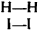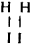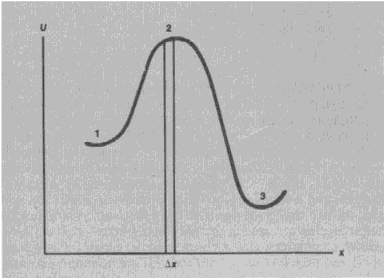# Activated Complex

(redirected from Activated Complex Theory)
Also found in: Medical, Acronyms.

## activated complex

[′ak·tə‚vād·əd ′käm·pleks]
(physical chemistry)
An energetically excited state which is intermediate between reactants and products in a chemical reaction. Also known as transition state.
McGraw-Hill Dictionary of Scientific & Technical Terms, 6E, Copyright © 2003 by The McGraw-Hill Companies, Inc.
The following article is from The Great Soviet Encyclopedia (1979). It might be outdated or ideologically biased.

## Activated Complex

the grouping of atoms at the decisive moment of an elementary chemical reaction. The concept of the activated complex is widely used in the theory of chemical reaction rates.

The course of an elementary reaction can be examined using the example of the gaseous bimolecular reaction of the formation of hydrogen iodide from hydrogen and iodine vapors:

(1) H2 = I2 = 2HI

The theory of quantum mechanics shows that as the H 2and I2 molecules approach within a distance comparable to molecular dimensions, as they repel each other with a force that increases rapidly as the distance decreases. The overwhelming majority of the collisions of H2 and I2 molecules in the gas mixture do not lead to a reaction, because the energy of the thermal movement of the molecules is insufficient to overcome the repulsion. In a certain very small fraction of the molecules, the intensity of thermal movement happens to be much higher than average; this makes it possible for the H2 and I2 molecules to approach each other so closely that new chemical bonds form between the H and I atoms, while the previously existing H—H and I—I chemical bonds are broken. The two newly formed HI molecules repel each other and therefore move apart; this completes the elementary reaction.

The transition from the bond arrangementto the arrangementtakes place gradually rather than suddenly:

as the H2 and I2 molecules come closer together, the H—H and I—I bonds weaken and at the same time the H—I bonds strengthen. Repulsion of H—H from I—I gives way to repulsion of H—I from H—I at the moment when the new bond arrangement begins to prevail over the old one. Thus, in the course of the elementary reaction, a configuration of atoms arises that is critical in the sense that, once it has been achieved, the further movement of the atoms proceeds unimpeded, without the need for an energy supply. The aggregate of atoms in this configuration is called an activated complex (H. Eyring, USA, 1935) or a transition state (M. G. Evans and M. Polanyi, England, 1935). For the reverse reaction,

(2) 2HI = H2 + I2

the arrangement of the atoms in the activated complex is the same as for the forward reaction (1), but the directions of movement of the atoms in the activated complexes in reactions (1) and (2) are opposite.

The energy relations in an elementary reaction can be schematically depicted by means of a graph in which the potential energy U of the reacting system is plotted as a function of the so-called reaction coordinate x, which describes the arrangement of the atoms with respect to each other.

Upon specifying a certain very small interval Δx (see Figure 1) and assuming that the configuration of the atoms corresponds to the activated complex, if the value of the coordinate x falls within this interval, one can introduce the concepts of the concentration of activated complexes for the forward reaction in a given reacting system c+ and of their lifetime τ. During time τ, there are, per unit volume, c+ forward reactions. Since the rate of the forward reaction r+ is the number of corresponding reactions per unit volume per unit time,

(3) r+ = c+

Since the interval Δξ is small, both c+ and τ are proportional to Ax, and thus their ratio is independent of the arbitrarily chosen value of Δξ. The values of c+ and τ are calculated by methods of statistical mechanics. These methods use a number of simplifying assumptions, the most important one being that the occurrence of the reaction does not disturb the statistical equilibrium distribution of the molecules over different states.Figure 1. (1) initial state, (2) activated complex, (3) final state

Equation (3) expresses the basic idea of the theoretical interpretation of reaction rates on the basis of the concept of activated complexes. It not only makes it possible to evaluate the dependence of the reaction rate on concentration of reagents, temperature, and other factors, but it also determines the absolute value of the reaction rate. Therefore, the method of activated complexes is often called the theory of absolute reaction rates. In a rather small number of reactions, the chemical bonds are rearranged with difficulty, and therefore achievement of the activated-complex configuration still does not guarantee that the elementary reaction will be implemented. In order to take account of these so-called nonadiabatic reactions, an additional factor, the transmission coefficient, is introduced into the right side of equation (3); for nonadiabatic reactions, this factor is much smaller than one.

The initial concepts of the method of activated complexes were explained above using the example of a homogeneous gas reaction, but this method is also used for the calculation of the rates of reactions in solutions, in reactions of heterogeneous catalysis, and generally in all cases where the transformation involves the necessity of a chance concentration of energy of thermal movement in amounts considerably exceeding the average energy of the molecules at a given temperature.

Comparison of the theory of absolute reaction rates with experimental data, as well as theoretical analysis of its premises, shows that this theory, although not completely accurate, is a good approximation, valuable by virtue of its simplicity.

### REFERENCE

Glasstone, S., K. J. Laidler, and H. Eyring. Teoriia absoliutnykh skorostei reaktsii. Moscow, 1948. (Translated from English.)

M. I. TEMKIN